# Lua编程

1.SciTE安装包

Github 下载地址：https://github.com/rjpcomputing/luaforwindows/releases

1.单行注释--

--这里是单行注释

2.多行注释

--[[

]]--

1.数据类型nil

lua中的空值类型，删除变量时将变量设置为nil值即可。没有声明的变量的值都是nil。

b = 1
print(b)
b = nil
print(b)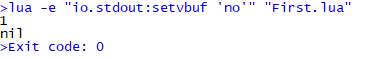2.数据类型boolean

if nil then
print(' ')
else
print(false)
end

if 1 then
print(true)
end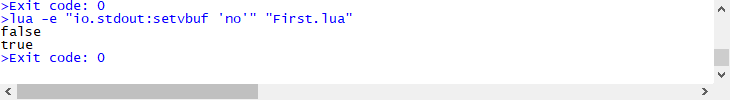3.数据类型number

lua中的数字都是number类型，可以理解为就是c中的double类型。number有如下写法：

print(2)
print(2.2)
print(2e3)
print(2.34e5)
print(3.1e-2)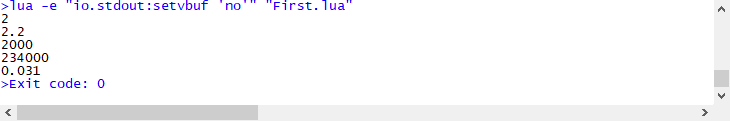4.数据类型string

print('我是字符串')
print("我是字符串")
print([[我是字符串]])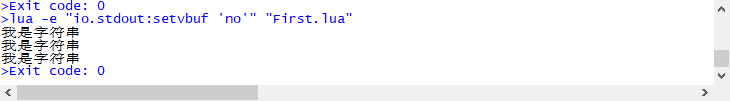字符串的拼接使用..，不能使用+号。

print('lua中的+号会自动将'..'字符串两边的字符串转化为数字')
print('2'..3)
print('2'+'3')
print(2+3)
print('2'+3)
print('2+3')
print('a'+3)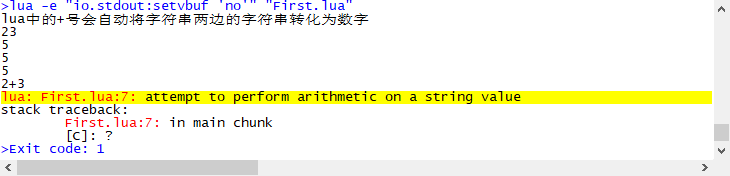使用#获得字符串的字节数。

print(#'aaa aa')
print(#'中文一个字符占用两个字节')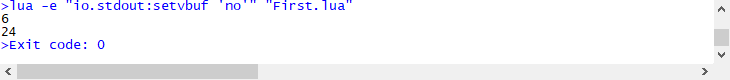5.数据类型table

tab1 = {}  --空表  {}构造表达式
tab2 = {key1='value1',key2='value2'}  --使用键值对的形式构造表（和map或dictionary等类似）
tab3 = {'value1','value2','value3'}  --直接给出表的值，默认索引为1,2,3,4...，和数组或list等的性质类似

print(tab1)  --直接打印表，打印的是表的地址
print(tab1.key)  --表中没有的键对应值为nil

print(tab2.key1)  --获取表中的某个索引对应值的方法一
print(tab2['key1'])  --获取表中某个索引对应值的方法二

print(tab3)  --没有给出索引的表默认索引是1,2,3,4...，不能使用方法一获取索引对应的值
print(tab3['1'])  --索引的类型是number，使用字符1进行取值不能取得值

--使用循环遍历表
for k,v in pairs(tab3) do
print(k..':'..v)
end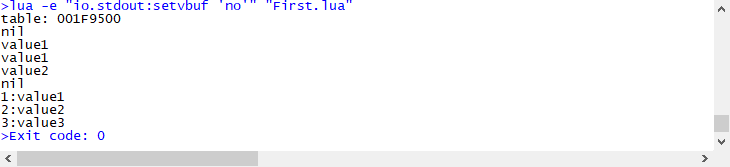表的添加值

tab = {}
tab.key1 = 'value1'
tab.key2 = 'value2'  --索引是字符串使用.赋值，使用中括号赋值会报错
tab = 100  --索引是number及其他类型使用中括号进行赋值，使用.赋值同样报错。nil不能作为索引。
tab[false] = 10

print(tab.key1)  --同样的，在获取值时也需要注意取值的方式
print(tab[key2])
print(tab)
print(tab[false])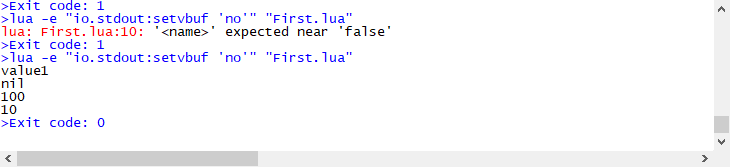6.数据类型function

function fact(n)
if n==1 then
return n
else
return n*(fact(n-1))
end
end

print(fact(4))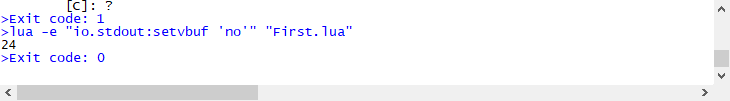函数同样可以作为值进行传递。

function fact(n)
if n==1 then
return n
else
return n*(fact(n-1))
end
end

print(fact(4))

fact2 = fact

print(fact2(5))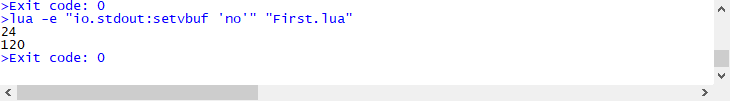函数也可以作为参数传递，甚至可以定义匿名函数（类似于委托）。

--定义测试函数
function testFun(tab,fun)
for k,v in pairs(tab) do
fun(k,v)
end
end

--定义表和参数中的函数
tab = {key1='value1',key2='value2'}
function f(k,v)
print(k..':'..v)
end

--调用测试函数
testFun(tab,f)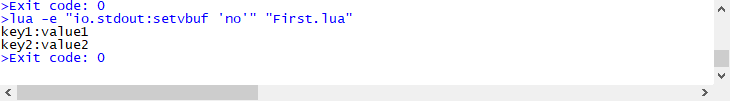--定义测试函数
function testFun(tab,fun)
for k,v in pairs(tab) do
fun(k,v)
end
end

--定义
tab = {key1='value1',key2='value2'}

--调用测试函数，以匿名函数的形式传递函数参数
testFun(tab,
function (k,v)
print(k..' '..v)
end
)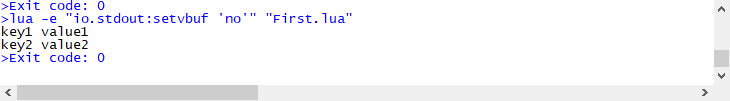8.数据类型userdata

userdata是用户自定义的数据类型，通常由C/C++创建。

1.全局变量和局部变量

lua中的变量不需要声明类型，默认情况下，变量都是全局变量。可以使用local关键字声明局部变量，局部变量只在当前代码块中起作用。一般情况下， 访问局部变量的速度更快。

function test()
a = 5
local b = 6
end

test()

print(a)
print(b)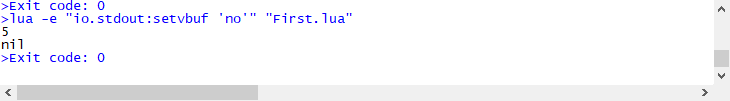2.变量的赋值

--可以单变量赋值，也可以多变量赋值
a = 1
a,b = 1,2
a,b,c = 1,'a',3

print(a,b,c)

--交换两个变量的值
a,b = b,a
print(a,b,c)

--交换三个变量的值
a,b,c = c,a,b
print(a,b,c)

--变量的个数多于值，多出来的变量会被自动忽略；值的个数多于变量，多出来的值会被自动忽略
c,d,e = 1,2
f,g = 4,5,6
print(c,d,e,f,g)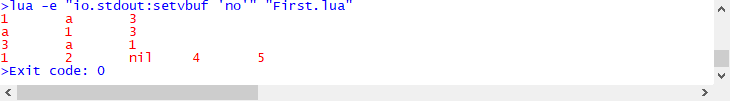3.循环

while循环。

i = 1
while i<=5 do
print(i)
i=i+1
end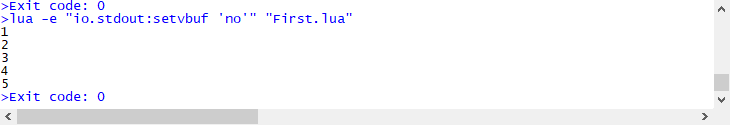for循环。之前也有用到过遍历表的for循环，类似于foreach的使用。

--i从1自增到5，每次自增1
for i=1,5 do
print(i)
end

--i从1自增到10，每次自增2
for i=1,10,2 do
print(i)
end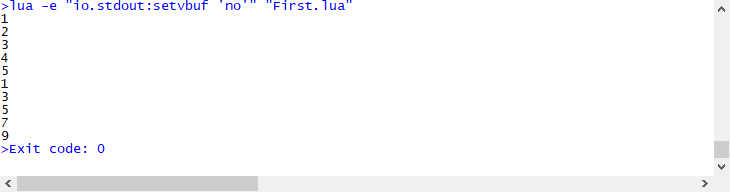repeat until循环。这个循环相当于do...while，但是和do...while有所不同。do...while是先执行一次do中的代码块，再判断while的逻辑语句，如果满足条件继续执行；repeat until是先执行一次until中的代码块，再判断until中的逻辑语句，如果不满足条件继续执行。

i = 1
repeat
print(i)
i=i+2
until i>10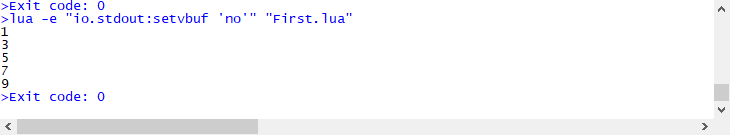4.分支结构

--if语句
if 0 then
print(0)
end

--if else语句
i = 1
if i<=0 then
print(0)
else
print(1)
end

--if else if ...语句
j = -2
if j <0 then
print(-1)
elseif j==0 then
print(0)
else
print(1)
end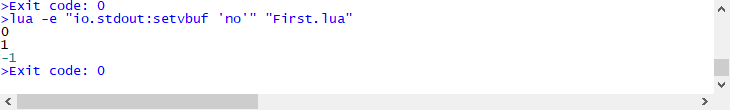注：循环结构和分支结构中的，while、for、if等语句后面跟上逻辑语句，这些逻辑语句可以使用括号括起来，也可以使用空格隔开，不使用括号，这里都没有使用括号。

5.函数

local function max(num1,num2)
if num1 < num2 then
return num2
else
return num1
end
end

print(max(3,-5))

--函数作为数据赋值
temp = max
print(temp(1,8))

myprint = function (param)
print('this is my print function '..param)
end

myprint('ww')

--函数作为参数传递
res = num1+num2
printFun(res)
end

--lua中的函数可以返回多个值
function temp()
return 10,20,30,40
end

res1,res2,res3,res4 = temp()
print(res1,res2,res3,res4)

--lua中的函数参数个数可变，如print函数
--...代表可变的参数，这些参数会被封装为一个名称为arg的表，使用表的方式访问这些参数，在使用for循环遍历表时arg会多一个值表示参数个数
--可变参数前可以有其他参数，但是可变参数一定在参数列表的最后，封装时只有可变参数部分会被封装到表arg中
function test(a,...)
for k,v in pairs(arg) do
print(v)
end
end

test(1,2,3)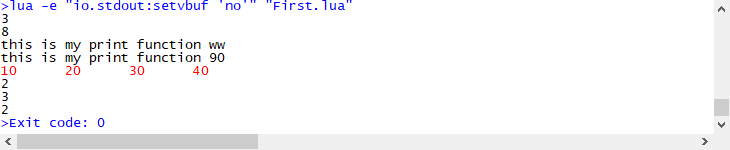六.lua中的运算符

1.算数运算符+、-、*、/、%、^（幂运算符在c#语言中不存在，c#中使用函数求幂）

2.关系运算符==、~=（不等于、 <、>、<=、>=

3.逻辑运算符and、or、not

print(3>2 and 4>3)
print(false or false)
print(not true)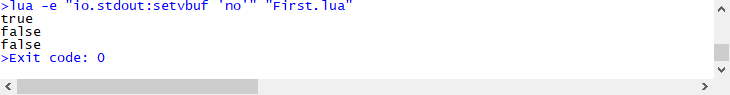七.lua中的常见API

1.string有关的API

a = 'hello\n\world my name is \'Micheal\''
print(a)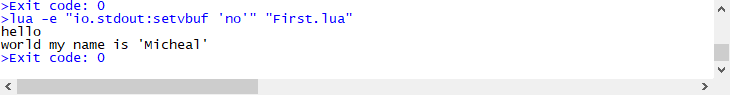string.upper 将字符串转换为全部大写 string.lower 将字符串转化为全部小写

str = 'Hello everybody'
str2 = string.upper(str)
print(str,str2)
str3 = string.lower(str)
print(str,str3)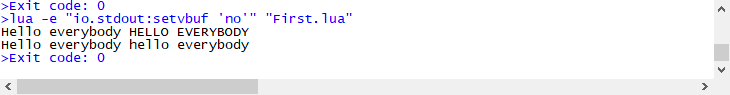string.gsub 将指定的字符替换为其他指定的字符

str = 'Hello everybody'
--将str中的字符e替换为字符8
str2 = string.gsub(str,'e','8')
print(str,str2)
--将str中的字符e替换为字符123，最多替换1次
str3 = string.gsub(str,'e','123',1)
print(str,str3)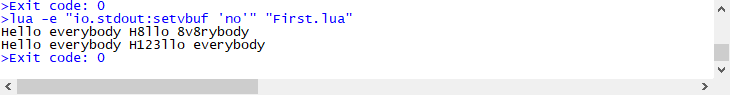string.find 查找指定字符的第一个索引

str = 'Hello everybody'

--从头查找'every'字符的索引（索引从1开始）
index = string.find(str,'every')
print(index)
--从第6个字符开始茶轴'o'字符的索引
index2 = string.find(str,'o',6)
print(index2)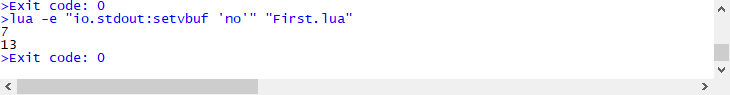string.reverse 反转字符串

str = 'Hello everybody'

str2 = string.reverse(str)
print(str2)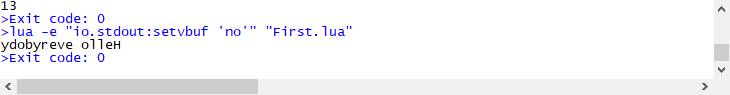string.format 字符串格式化输出（未知number使用%d代替，未知string使用%s代替，其他代替格式可以自行查阅）

num1 = 5
num2 = 10
str = string.format('加法：%d+%d=%d',num1,num2,num1+num2)
print(str)
date,month,year = 30,1,2021
d = string.format('日期：%02d/%02d/%03d',date,month,year)
print(d)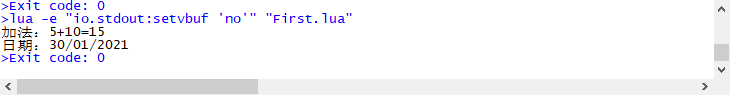string.char 将指定的数字转化为对应字符 string.byte 将字符转化为数字（默认转化字符串第一个字符，也可以指定转化第几个字符）

print(string.char(97,98,99,100))
print(string.byte('abcd'))
print(string.byte('abcd',3))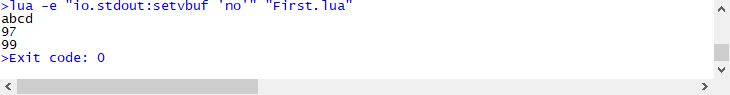string.len 获得指定字符串的长度（和#的结果相同）

print(string.len('我有一个梦想'))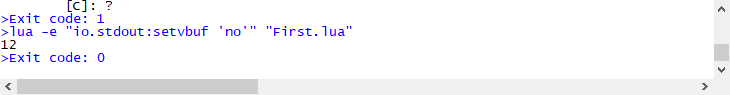string.rep 得到指定字符串重复指定次后的字符串

print(string.rep('我有一个梦想',5))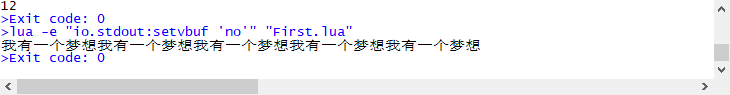string.gmatch 正则表达式匹配，返回一个迭代器

for word in string.gmatch('Hello lua user','%a+') do
print(word)
end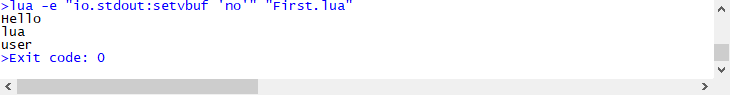2.table有关的API

lua中array本质上是table。因为table是动态的，因此lua中的array也是动态的。值得注意的是，table中的默认索引是从1开始的，因此array中的默认下标也是从1开始的。下面是一个二维数组的例子：

array = {}
for i=1,4 do
array[i] = {}
for j=1,3 do
array[i][j]=i*j
end
end

for i=1,4 do
for j=1,3 do
print(array[i][j])
end
end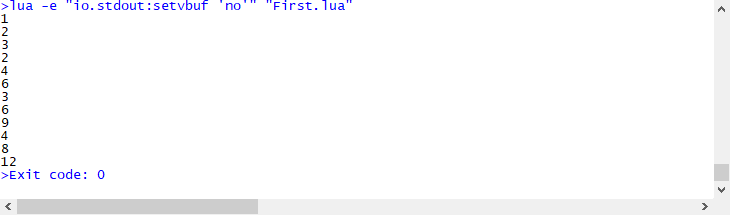数组的遍历。

array = {'lua','c#','java'}

--迭代函数一pairs：遍历table中的key和value
for k,v in pairs(array) do
print(k,v)
end

--迭代函数二ipairs：从索引1开始，递增遍历，遇到nil就停止
for k,v in ipairs(array) do
print(k,v)
end

array = nil

for k,v in ipairs(array) do
print(k,v)
end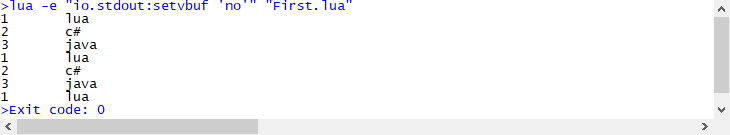table = {'a','b','c'}
newtable = table

print(table)
print(newtable)

newtable = 'd'

print(table)
print(newtable)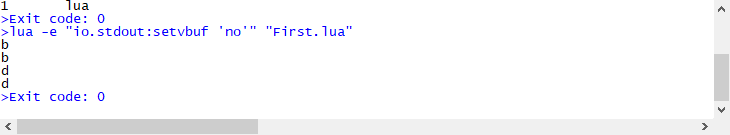表的数据拼接。

table1 = {'a','b','c'}

--直接拼接
str = table.concat(table1)
print(str)

--使用,间隔开拼接的数据
str = table.concat(table1,',')
print(str)

--使用,间隔开数据，指定拼接的索引
str = table.concat(table1,',',2,3)
print(str)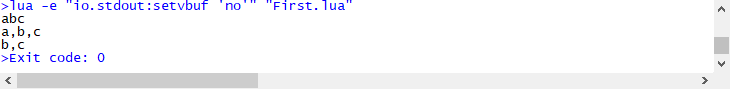表的数据插入和移除。

table1 = {'lua','c#','java','php'}
print(table.concat(table1,','))

--直接插入到末尾
table.insert(table1,'javascript')
print(table.concat(table1,','))

--指定插入到哪一位，后面的数据依次向后移动一位
table.insert(table1,2,'c++')
print(table.concat(table1,','))

--移除表一位数据
table.remove(table1)
print(table.concat(table1,','))

--移除指定位置的数据
table.remove(table1,3)
print(table.concat(table1,','))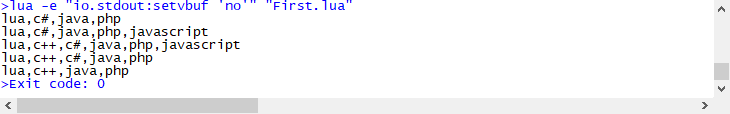table1 = {'lua','c#','java','php','c++','javascript'}
print(table.concat(table1,','))

--排序，按照ASCII码表顺序排列
table.sort(table1)
print(table.concat(table1,','))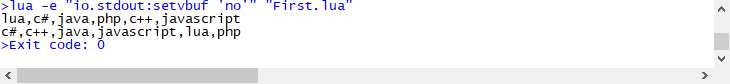1.模块与包

lua中的模块相当于c#的命名空间或java的包。

--定义模块，这个模块保存为文件Module.luavar = 'movin'

func1 = function()
print('这是一个函数')
end

return module
--引入模块
require 'Module'

--使用模块中的变量
print(var)
func1()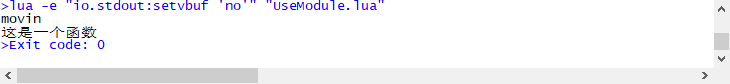lua中的包是指使用C包，lua和C很容易结合，lua就是使用C写成的。

2.元表

lua提供了元表允许我们改变table的行为，每种行为关联了对应的元方法。

mytable = {'lua','java','c#','c++'}  --普通表
mymetatable = {}  --元表  拓展了普通表的行为
mytable = setmetatable(mytable,mymetatable)  --关联元表和普通表

1)__index元方法，这是metatable中最常用的键。当通过键访问table的时候，如果这个键没有值，那么lua就会寻找该table的metatable中的__index键。如果__index包含一个表格，lua会在表中查找相应的键，如果__index包含一个函数，lua就会调用那个函数。

mytable = {'lua','java','c#','c++'}

mymetatable = {
__index=function (tab,key)
return 'javascript'
end
}

mytable = setmetatable(mytable,mymetatable)

print(mytable)
print(mymetatable)  --表和元表关联，但是两个表并不相同，元表是表的拓展
print(mytable)  --存在的键值
print(mymetatable)
print(mytable)  --不存在的键值，调用元表中__index键对应的函数
print(mymetatable)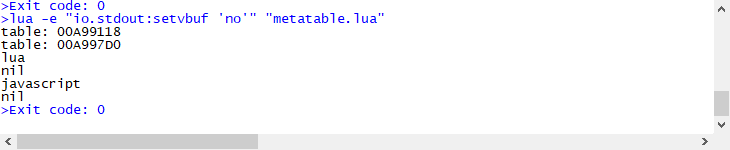2）__newindex元方法，当对表的新索引进行赋值时会起作用，并且会取消赋值操作。

mytable = {'lua','java','c#','c++'}

mymetatable = {
__newindex = function (tab,key,value)
print(string.format('我们要修改的key为%s，value为%s',key,value))
end
}

mytable = setmetatable(mytable,mymetatable)

--下面这些操作都不会触发__newindex对应的方法
mytable = 'javascript'
print(table.concat(mytable,'  '))
table.insert(mytable,'lua')
print(table.concat(mytable,'  '))
table.insert(mytable,3,'c')
print(table.concat(mytable,'  '))
table.remove(mytable,1)
print(table.concat(mytable,'  '))
table.remove(mytable)
print(table.concat(mytable,'  '))

mytable = 'lua'  --对新索引进行赋值时会调用__newtable对应的方法，而且不会进行赋值操作
print(table.concat(mytable,'  '))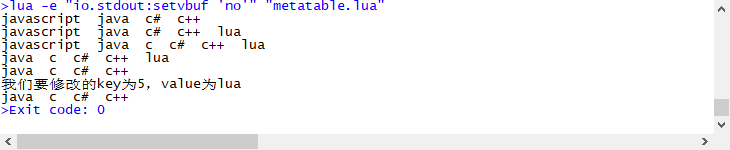mytable = {'lua','java','c#','c++'}

mymetatable = {
__newindex = function (tab,key,value)
rawset(tab,key,value)  --由于调用了__newindex对应的函数后不会进行赋值，若想赋值，可以使用rawset函数（如果直接赋值会产生死循环）
--mytable = 'lua'  这里采用这种方式赋值会产生死循环
end
}

mytable = setmetatable(mytable,mymetatable)

mytable = 'lua'
print(table.concat(mytable,'  '))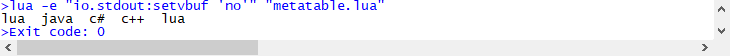mytable = {'lua','java','c#','c++'}

newtable = {}
mymetatable = {
__newindex = newtable  --__newindex对应的是一个表
}

mytable = setmetatable(mytable,mymetatable)

mytable = 'javascript'   --设置不存在的索引的值时会设置到__newindex对应的表中

print(mytable)
print(newtable)  --在__newindex对应的表中，索引值还是5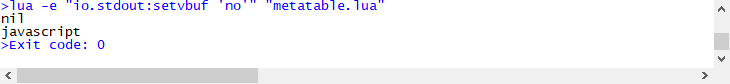3）操作符元方法。

mytable = {'lua','java','c#','c++'}

mymetatable = {
for k,v in pairs(newtab) do
table.insert(tab,v)
end

return tab
end
}

mytable = setmetatable(mytable,mymetatable)

newtable = {'python','php'}

print(table.concat(mytable+newtable,' '))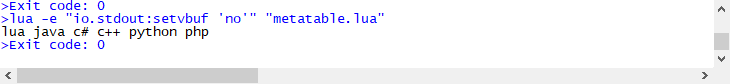4）__call元方法

mytable = {'lua','java','c#','c++'}

mymetatable = {
__call = function(tab,arg)
print(arg)
end
}

mytable = setmetatable(mytable,mymetatable)

mytable('我是大帅比')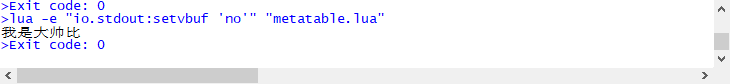5）__tostring元方法

mytable = {'lua','java','c#','c++'}

mymetatable = {
__tostring = function(tab)
str = ''
for k,v in pairs(tab) do
str = str..k..' '..v..','
end
return str
end
}

mytable = setmetatable(mytable,mymetatable)

print(mytable)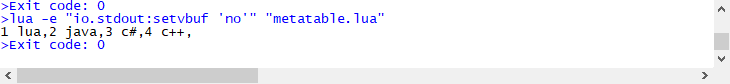3.协程

--定义协同函数
co = coroutine.create(
function (a,b)  --必须是匿名函数
print(a+b)
end
)

--运行协同函数
coroutine.resume(co,20,40)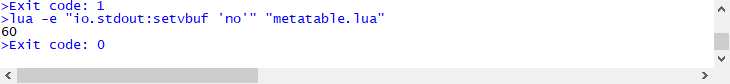方式二：

--定义协同函数
co = coroutine.wrap(
function (a,b)  --必须是匿名函数
print(a+b)
end
)

--运行协同函数
co(20,40)协程的挂起和继续运行。

--定义协同函数
co = coroutine.create(
function (a,b)
print(a+b)
coroutine.yield()  --挂起协同函数
print(a-b)
end
)

--运行协同函数
coroutine.resume(co,20,40)

--这句代码的位置在运行协同函数的代码后，在重启协同函数的代码前，因此挂起协同函数后会运行这段代码
print("I'm here")

--继续运行协同函数
coroutine.resume(co)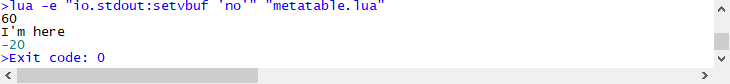协同程序的返回值。

co = coroutine.create(
function (a,b)
return a+b,a-b
end
)

--协同函数默认有一个boolean类型的返回值表示是否启动成功，自己定义的其他返回值作为第二个、第三个......等返回值
res1,res2,res3 = coroutine.resume(co,20,40)

print(res1,res2,res3)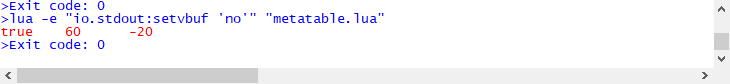co = coroutine.create(
function (a,b)
print('........................')
coroutine.yield(a+b,a-b)
print('........................')
return a*b,a/b
end
)

--当协同函数中间被暂停时，可以分阶段返回不同的返回值，使当前阶段暂停的yield函数括号中的参数作为当前阶段的返回值
res1,res2,res3 = coroutine.resume(co,20,40)

print(res1,res2,res3)

--最后一个运行阶段的返回值为return返回的内容
res4,res5,res6 = coroutine.resume(co)

print(res4,res5,res6)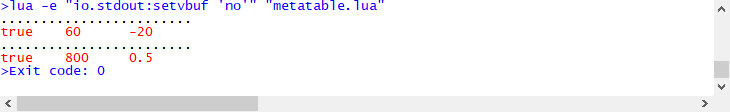协程的状态（3种）。

co = coroutine.create(
function (a,b)
print(coroutine.status(co))  --running运行
print('........................')
coroutine.yield()
print('........................')
print(coroutine.status(co))  --running运行
end
)

print(coroutine.status(co))  --suspended暂停
coroutine.resume(co,20,40)
print(coroutine.status(co))  --suspended暂停
coroutine.resume(co)
print(coroutine.status(co))  --dead死亡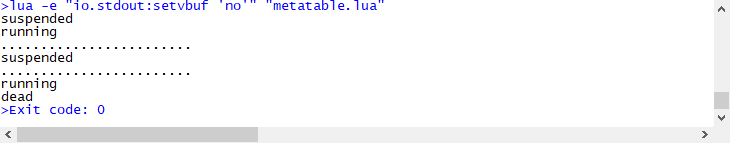获取正在运行的协程。

co = coroutine.create(
function (a,b)
print(coroutine.running())  --获取正在运行的协程
end
)

coroutine.resume(co,20,40)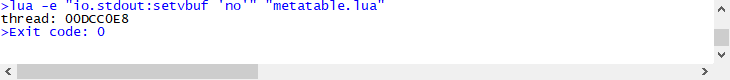4.文件I/O

f = io.open('iotest.txt','r')  --打开文件
io.input(f)  --创建输入流
io.close()  --关闭流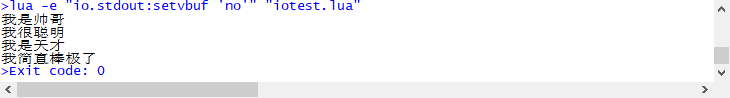open函数的第一个参数是文件的相对地址和名称，第二个参数是可选参数，对应打开方式。打开方式有：r（只读，文件必须存在）、w（只写，写入的文件原有数据会被清空，文件不存在会自动创建文件）、a（以附加的方式打开只写文件。若文件不存在，则会建立该文件，如果文件存在，写入的数据会被加到文件尾，即文件原先的内容会被保留）、r+（以可读写方式打开文件，该文件必须存在）、w+（打开可读写文件，若文件存在则文件长度清为零，即该文件内容会消失。若文件不存在则建立该文件）、a+（与a类似，但此文件可读可写）、b（二进制模式，如果文件是二进制文件，可以加上b）

f = io.open('iotest.txt','a')  --打开文件，a为追加的形式只写，w为清空后只写
io.output(f)  --创建输出流
print(io.write(''))  --write函数写入内容，返回值代表是否写入成功
io.close()  --关闭流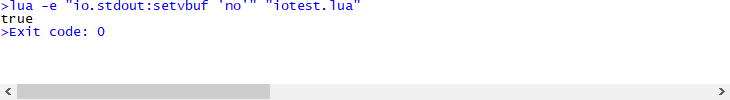f = io.open('iotest.txt','r')
io.input(f)
io.close()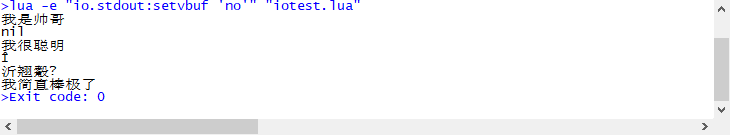完全模式。完全模式下，可以同时处理多个文件。

f = io.open('iotest.txt','r')

file.close()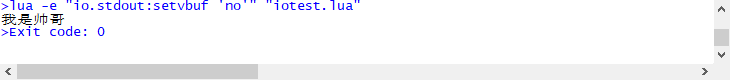5.lua实现面向对象编程

lua中并没有直接实现面向对象编程，但是可以使用表间接实现面向对象。

--定义一个人的对象
person = {name='movin',age=18}

person.eat = function ()
print(person.name..'在吃饭')
end

person.eat()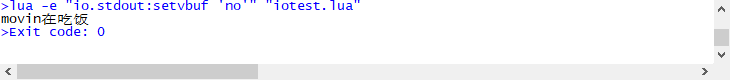优化面向对象的实现。

--定义一个人的对象
person = {name='movin',age=18}

--将对象自身作为变量传递，否则这里对象的名称不能修改
person.eat = function (self)
print(self.name..'在吃饭')
end

person.eat(person)--定义一个人的对象
person = {name='movin',age=18}

--使用冒号定义和调用，不用传递self参数，其中self就指代调用者
function person:eat()
print(self.name..'在吃饭')
end

person:eat()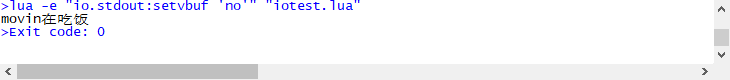根据模板创建新对象。

Person = {name='movin',age=18}

function Person:eat()
print(self.name..'在吃饭')
end

--创建新的对象的new方法
function Person:new()
local t = {}
--使用元表的__index元方法指向Person对象
setmetatable(t,{__index=self})
return t
end

person1 = Person:new()
person2 = Person:new()

print(person1.name)
person2:eat()

--修改对象中的属性值相当于在对象中设置了新值，再查找这个索引对应的值时就不会在Person中查找，相当于实现了修改属性和重写方法的效果
person1.name = 'ww'

print(person1.name)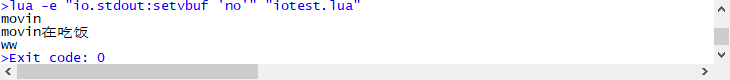__index元方法相当于实现了继承，元表相当于父表。当子表中没有某个属性或方法时，从父表中查找；当子表中重新赋值了某些属性或重写了某些方法时，就直接从子表中调用。

posted @ 2021-01-30 12:40  movin2333  阅读(143)  评论(0编辑  收藏  举报